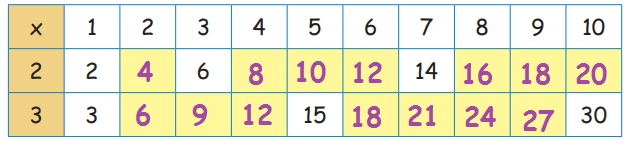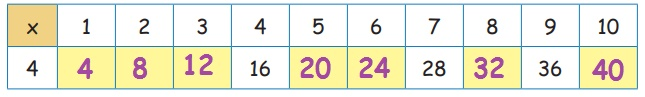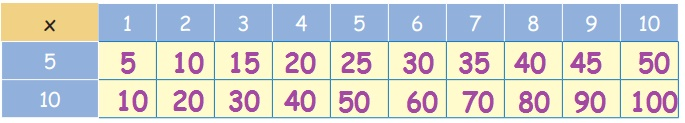Home | | Maths 3rd Std | Construction of multiplication tables of 2, 3, 4, 5, & 10

# Construction of multiplication tables of 2, 3, 4, 5, & 10

Construction of multiplication tables of 2, 3, 4, 5, & 10

Construction of multiplication tables of 2, 3, 4, 5, & 10

Multiplication table 2Multiplying by 2:2 + 2 + 2 + 2 = 8

2 leaves in 4 groups is 8.

This can be written as 2 × 4 = 8

4 times 2 is 8Activity :Exercise

Fill in the boxes:

2 × 6 = 12

9 × 2 = 18

3 × 2 = 6

7 × 2 = 14

2 × 5 = 10

2 × 2 = 4

8 × 2 = 16

4 × 2 = 8

2 × 3 = 6

Multiplication table 3Activity :

Shall we say the multiples of 3.Multiples of 3 = 3, 6, 9, 12, 15, 18

Exercise

1. Fill in the following tables:2. Fill in the boxes:

6×3= 18

10×3= 30

4×3= 12

5×3= 15

3×6= 18

3×10= 30

3×3= 9

8×3= 24

3×4= 12

3 ×3=9

3 =6

3 = 27

Multiplication table 4Exercise

1. Complete the table.2. If there are 4 toys in a box, how many toys will be there in 5 boxes?4 × 5 = 20

3. Fill in the boxes.

3 × 4 = 12

5 × 4 = 20

7 × 4 = 28

9 × 4 = 36

6 × 4 = 24

4 × 3 = 12

4 × 4 = 16

10 × 4 = 40

Multiplication table 5Exercise

1. Fill in the blanks:

2 × 5 = 10

4 × 5 = 20

6 × 5 = 30

9 × 5 = 45

10 × 5 = 50

2.If there are 6 roses in a vase, how many roses will be there in 6 vases?

6 × 6 = 36

Multiplication table 10Exercise

Complete the following table:Tags : Numbers | Term 2 Chapter 1 | 3rd Maths , 3rd Maths : Term 2 Unit 1 : Numbers
Study Material, Lecturing Notes, Assignment, Reference, Wiki description explanation, brief detail
3rd Maths : Term 2 Unit 1 : Numbers : Construction of multiplication tables of 2, 3, 4, 5, & 10 | Numbers | Term 2 Chapter 1 | 3rd Maths